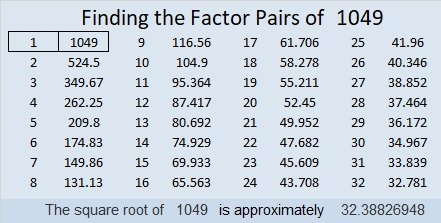# 1049 and Level 6

Find the Factors Puzzles are always solved using logic. Can you see the logic needed to solve this one?Print the puzzles or type the solution in this excel file: 12 factors 1044-1053

Here are a few facts about the number 1049:

• 1049 is a prime number.
• Prime factorization: 1049 is prime.
• The exponent of prime number 1049 is 1. Adding 1 to that exponent we get (1 + 1) = 2. Therefore 1049 has exactly 2 factors.
• Factors of 1049: 1, 1049
• Factor pairs: 1049 = 1 × 1049
• 1049 has no square factors that allow its square root to be simplified. √1049 ≈ 32.38827

How do we know that 1049 is a prime number? If 1049 were not a prime number, then it would be divisible by at least one prime number less than or equal to √1049 ≈ 32.4. Since 1049 cannot be divided evenly by 2, 3, 5, 7, 11, 13, 17, 19, 23, 29 or 31, we know that 1049 is a prime number.1049 is also the sum of three consecutive prime numbers:
347 + 349 + 353 = 1049

32² + 5² = 1049 so 1049 is the hypotenuse of a Pythagorean triple:
320-999-1049 calculated from 2(32)(5), 32² – 5², 32² + 5²

Here’s another way we know that 1049 is a prime number: Since its last two digits divided by 4 leave a remainder of 1, and 32² + 5² = 1049 with 32 and 5 having no common prime factors, 1049 will be prime unless it is divisible by a prime number Pythagorean triple hypotenuse less than or equal to √1049 ≈ 32.4. Since 1049 is not divisible by 5, 13, 17, or 29, we know that 1049 is a prime number.

This site uses Akismet to reduce spam. Learn how your comment data is processed.